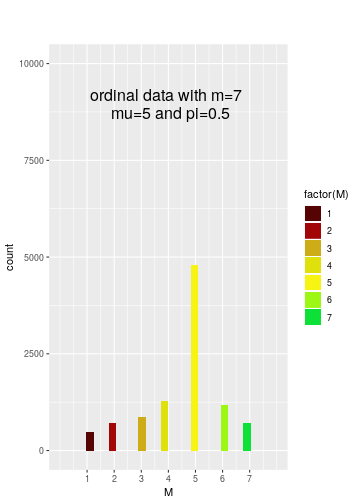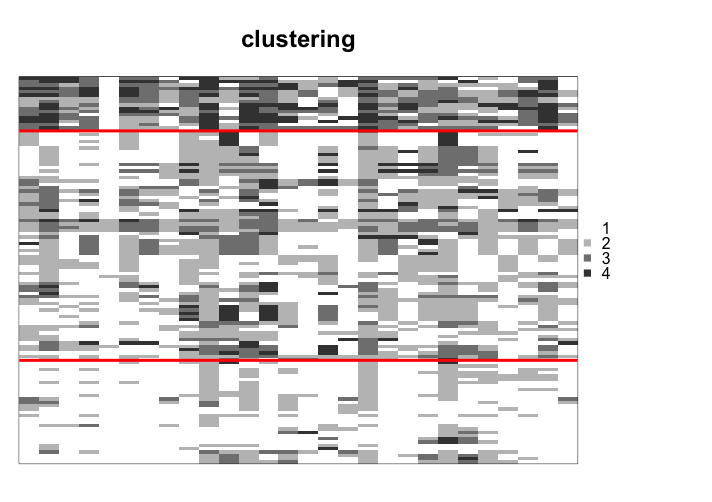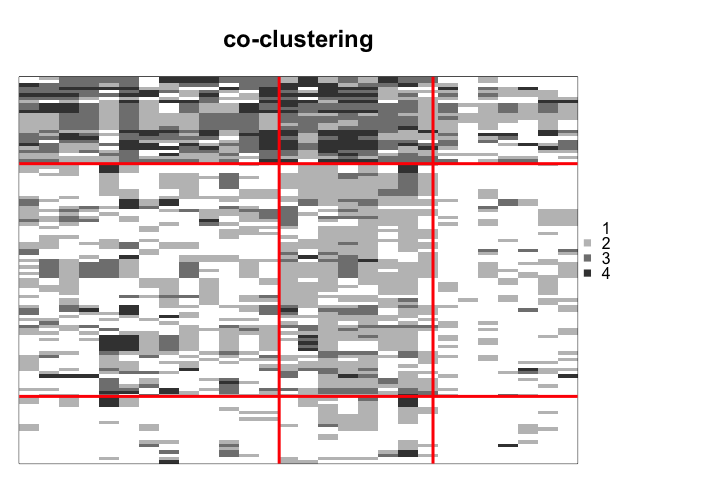## Description

ordinalClust is an R package to perform classification, clustering and co-clustering of ordinal data. Furthermore, it can handle different numbers of levels and missing values. The ordinal data is considered to follow a BOS distribution [@biernacki16], which is specific to this kind of data. The Latent Block Model is used for performing co-clustering [@jacques17].

## Installation

``````set.seed(1)
``````
``````library(ordinalClust)
``````

## Datasets

The package contains real datasets created from [@Anota17]. They relate to quality of life questionnaires for patients affected by breast cancer.

• dataqol is a dataframe with 121 lines such that each line represents a patient and the columns contain information about the patient:

• Id: patient Id
• q1-q28: responses to 28 questions with the number of levels equal to 4
• q29-q30: responses to 2 questions with the number of levels equal to 7
• dataqol.classif is a dataframe with 40 lines such that a line represents a patient and the columns contain information about the patient:

• Id: patient Id
• q1-q28: responses to 28 questions with the number of levels equal to 4
• q29-q30: responses to 2 questions with the number of levels equal to 7
• death: if the patient survived (1) or not (2).

## Univariate Ordinal Data Simulation

To simulate a sample of ordinal data following the BOS distribution, the function pejSim is used.

### Basic example code

This snippet of code creates a sample of ordinal data with 7 levels that follows a BOS distribution parameterized by mu=5 and pi=0.5:

``````m=7
nr=10000
mu=5
pi=0.5

probaBOS=rep(0,m)
for (im in 1:m) probaBOS[im]=pejSim(im,m,mu,pi)
M <- sample(1:m,nr,prob = probaBOS, replace=TRUE)
``````

### Plotting

To plot the resulting distribution, the ggplot2 library can be used.## Performing clustering

In this section, clustering is executed using the dataqol dataset. The purpose of performing clustering is to highlight the structure through the matrix rows.

### Example code

``````set.seed(0)

library(ordinalClust)
data("dataqol")

M <- as.matrix(dataqol[,2:29])

m = 4

krow = 3

nbSEM=100
nbSEMburn=90
nbindmini=2
init = "randomBurnin"
percentRandomB = c(30)

object <- bosclust(x=M,kr=krow, m=m, nbSEM=nbSEM,
nbSEMburn=nbSEMburn, nbindmini=nbindmini,
percentRandomB=percentRandomB, init=init)
``````

### Plotting the result

``````plot(object)
``````## Performing co-clustering

### Example code

In this example, co-clustering is performed using the dataqol dataset. In this case, the interest in performing co-clustering is to detect an internal structure throughout the rows and columns of the data.

``````set.seed(0)

library(ordinalClust)

data("dataqol")

M <- as.matrix(dataqol[,2:29])

# defining different number of categories:
m=4

# defining number of row and column clusters
krow = 3
kcol = 3

# configuration for the inference
nbSEM=100
nbSEMburn=90
nbindmini=2
init = "randomBurnin"
percentRandomB = c(30, 30)

# Co-clustering execution
object <- boscoclust(x = M,kr = krow, kc = kcol, m = m,
nbSEM = nbSEM, nbSEMburn = nbSEMburn,
nbindmini = nbindmini, init = init,
percentRandomB = percentRandomB)
``````

### Plotting the result

This snippet of code shows how to visualize the resulting co-clustering, using the plot function:

``````plot(object)
``````## Performing classification

In this section, the dataset dataqol.classif is used. It contains the responses to a questionnaire by 40 patients affected by breast cancer. Furthermore, a column labeled death indicates whether the patient died from the disease (2) or not (1). The aim of this section is to predict the classes of a validation dataset from a training dataset.

### Choosing a good kc parameter with cross-validation

The classification function bosclassif provides two classiﬁcation models. The ﬁrst model, (chosen by the option kc=0), is a multivariate BOS model with the assumption that, conditional to the class of the observations, the features are independent. The second model is a parsimonious version of the ﬁrst model. Parsimony is introduced by grouping the features into clusters (as in co-clustering) and assuming that the features of a cluster have a common distribution. The number L of clusters of features is defined with the option kc=L. In practice, L can be chosen by cross-validation, as shown in the following example:

``````set.seed(1)

library(ordinalClust)
data("dataqol.classif")

M <- as.matrix(dataqol.classif[,2:29])

# creating the classes values
y <- as.vector(dataqol.classif\$death)

# sampling datasets for training and to predict
nb.sample <- ceiling(nrow(M)*7/10)
sample.train <- sample(1:nrow(M), nb.sample, replace=FALSE)

M.train <- M[sample.train,]
M.validation <- M[-sample.train,]
nb.missing.validation <- length(which(M.validation==0))

y.train <- y[sample.train]
y.validation <- y[-sample.train]

# number of classes to predict
kr <- 2

# configuration for SEM algorithm
nbSEM=200
nbSEMburn=175
nbindmini=2
init="randomBurnin"
percentRandomB = c(50, 50)

# different kc to test with cross-validation
kcol <- c(0,1,2,3)
m <- 4

# matrix that contains the predictions for all different kc
preds <- matrix(0,nrow=length(kcol),ncol=nrow(M.validation))

for(kc in 1:length(kcol)){
res <- bosclassif(x=M.train, y=y.train,
kr=kr, kc=kcol[kc], m=m,
nbSEM=nbSEM, nbSEMburn=nbSEMburn,
nbindmini=nbindmini, init=init, percentRandomB=percentRandomB)

new.prediction <- predict(res, M.validation)
preds[kc,] <- new.prediction@zr_topredict

}

preds = as.data.frame(preds)
row.names <- c()
for(kc in kcol){
name= paste0("kc=",kc)
row.names <- c(row.names,name)
}
rownames(preds)=row.names
``````

### Computing the sensitivity and specificity rates for each kc

``````library(caret)

actual <- y.validation -1

specificities <- rep(0,length(kcol))
sensitivities <- rep(0,length(kcol))

for(i in 1:length(kcol)){
prediction <- unlist(as.vector(preds[i,])) -1
u <- union(prediction, actual)
conf_matrix<-table(factor(prediction, u),factor(actual, u))
sensitivities[i] <- recall(conf_matrix)
specificities[i] <- specificity(conf_matrix)
}

sensitivities
``````
``````##  1.0 0.5 1.0 1.0
``````
``````specificities
``````
``````##  0.125 0.625 0.375 0.125
``````

## Handling different numbers of levels

The package can deal with ordinal data with different numbers of levels. In this section, we show how to introduce these kinds of datasets in a co-clustering context.

### Example code

In this example, co-clustering is performed using the dataset dataqol, by including the questions with 4 levels, and questions with 7 levels. The function boscoclustMulti is executed, which might take a few minutes.

``````set.seed(0)

library(ordinalClust)

data("dataqol")

M <- as.matrix(dataqol[,2:31])

# defining different number of categories:
m=c(4,7)

# defining number of row and column clusters
krow = 3
kcol = c(3,1)

# configuration for the inference
nbSEM=50
nbSEMburn=40
nbindmini=2
init='random'

d.list <- c(1,29)

# Co-clustering execution
object <- boscoclust(x=M,kr=krow,kc=kcol,m=m, idx_list=d.list,
nbSEM=nbSEM,nbSEMburn=nbSEMburn,
nbindmini=nbindmini, init=init)
``````